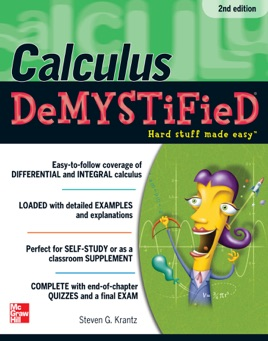• 10,99 €

## Descrizione dell’editore

Calculate this: learning CALCULUS just got a whole lot easier!

Stumped trying to understand calculus? Calculus Demystified, Second Edition, will help you master this essential mathematical subject.

Written in a step-by-step format, this practical guide begins by covering the basics--number systems, coordinates, sets, and functions. You'll move on to limits, derivatives, integrals, and indeterminate forms. Transcendental functions, methods of integration, and applications of the integral are also covered. Clear examples, concise explanations, and worked problems make it easy to understand the material, and end-of-chapter quizzes and a final exam help reinforce key concepts.

It's a no-brainer! You'll get:
Applications of the derivative and the integral

Rules of integration

Coverage of improper integrals

An explanation of calculus with logarithmic and exponential functions

Details on calculation of work, averages, arc length, and surface area

Simple enough for a beginner, but challenging enough for an advanced student, Calculus Demystified, Second Edition, is one book you won't want to function without!